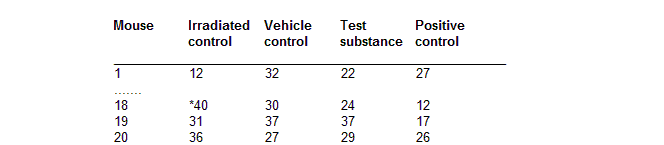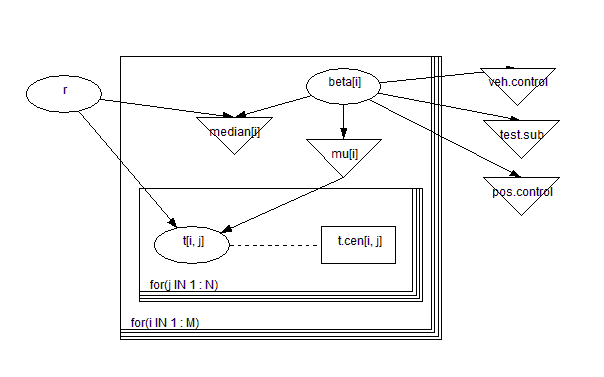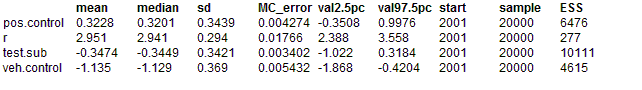#### Mice: Weibull regression

Dellaportas and Smith (1993) analyse data from Grieve (1987) on photocarcinogenicity in four groups, each containing 20 mice, who have recorded a survival time and whether they died or were censored at that time. A portion of the data, giving survival times in weeks, are shown below. A * indicates censoring.The survival distribution is assumed to be Weibull. That is

f (ti, zi) = reβzi tir - 1exp(-eβzitir)

where ti is the failure time of an individual with covariate vector zi and β is a vector of unknown regression coefficients. This leads to a baseline hazard function of the form

λ0(ti) = rtir - 1

Setting μi = eβzi gives the parameterisation

ti ~ Weibull(τ, μi)

For censored observations, the survival distribution is a truncated Weibull, with lower bound corresponding to the censoring time. The regression β coefficients were assumed a priori to follow independent Normal distributions with zero mean and ``vague'' precision 0.0001. The shape parameter r for the survival distribution was given a Gamma(1, 0.0001) prior, which is slowly decreasing on the positive real line.

Median survival for individuals with covariate vector zi is given by mi = (log2e-βzi)1/r

The appropriate graph and BUGS language are below, using an undirected dashed line to represent a logical range constraint.model
{
for(i in 1 : M) {
for(j in 1 : N) {
t[i, j] ~ dweib(r, mu[i])C(t.cen[i, j],)
}
mu[i] <- exp(beta[i])
beta[i] ~ dnorm(0.0, 0.001)
median[i] <- pow(log(2) * exp(-beta[i]), 1/r)
}
#r ~ dexp(0.001)
r ~ dunif(0.1, 10)
veh.control <- beta - beta
test.sub <- beta - beta
pos.control <- beta - beta
}

We note a number of tricks in setting up this model. First, individuals who are censored are given a missing value in the vector of failure times t, whilst individuals who fail are given a zero in the censoring time vector t.cen (see data file listing below). The truncated Weibull is modelled using C(t.cen[i],) to set a lower bound. Second, we set a parameter beta[j] for each treatment group j.The contrasts beta[j] with group 1 (the irradiated control) are calculated at the end. Alternatively, we could have included a grand mean term in the relative risk model and constrained beta to be zero.
##### Data
``` list(t = structure(.Data =       c(12, 1, 21, 25, 11, 26, 27, 30, 13, 12, 21, 20, 23, 25, 23, 29, 35, NA, 31, 36,          32, 27, 23, 12, 18, NA, NA, 38, 29, 30, NA, 32, NA, NA, NA, NA, 25, 30, 37, 27,          22, 26, NA, 28, 19, 15, 12, 35, 35, 10, 22, 18, NA, 12, NA, NA, 31, 24, 37, 29,          27, 18, 22, 13, 18, 29, 28, NA, 16, 22, 26, 19, NA, NA, 17, 28, 26, 12, 17, 26),         .Dim = c(4, 20)),      t.cen = structure(.Data =       c( 0, 0, 0, 0, 0, 0, 0, 0, 0, 0, 0, 0, 0, 0, 0, 0, 0, 40, 0, 0,          0, 0, 0, 0, 0, 40, 40, 0, 0, 0, 40, 0, 40, 40, 40, 40, 0, 0, 0, 0,          0, 0, 10, 0, 0, 0, 0, 0, 0, 0, 0, 0, 24, 0, 40, 40, 0, 0, 0, 0,          0, 0, 0, 0, 0, 0, 0, 20, 0, 0, 0, 0, 29, 10, 0, 0, 0, 0, 0, 0),         .Dim = c(4, 20)),      M = 4, N = 20) ```
##### Inits for chain 1
``` list(beta = c(-1, -1, -1, -1), r = 1) ```

##### Inits for chain 2
``` list(beta = c(-5,-5,-5,-5), r = 2) ```
Results